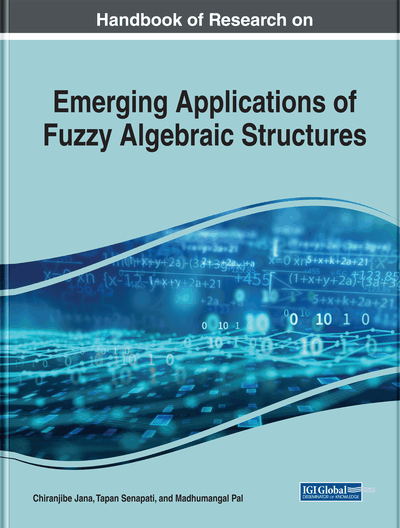On More Generalized Fuzzy Interior Ideals in Semigroup

DOI: 10.4018/978-1-7998-0190-0.ch010
Available
\$37.50
No Current Special Offers

Abstract

In this chapter, the authors introduce the concepts of (∈,∈∨qkδ)-fuzzy interior ideal in semigroup, which is a generalisation of (∈,∈∨qk)-fuzzy interior ideal and (∈,∈∨q)-fuzzy interior ideal in semigroup, and investigate some of its properties. They give example to show that (∈,∈∨qkδ)-fuzzy interior ideal in semigroup is not an (∈,∈∨qk)-fuzzy interior ideal and (∈,∈∨q)-fuzzy interior ideal in semigroup. They show that every (∈,∈∨qkδ)-fuzzy ideal is an (∈,∈∨qkδ)-fuzzy interior ideal, but the converses are not true in general.
Chapter Preview
Top

1 Introduction

In 1965, the concept of a fuzzy set was first introduced by, Zadeh in his important paper (Zadeh, 1965). This inspirational paper has opened up new awareness and application in a wide range of scientific fields. After the Zadeh's fuzzy sets many researches convoyed the researches on the generalizations of the of fuzzy sets ideas, with huge applications in computer science, artificial intelligence, control engineering, expert, robotics, automat theory, finite state machine, graph theory logics and many branches of pure and applied mathematics. Investigations of fuzzy groups and fuzzy semigroups have a long convention, starting with the opening of fuzzy period. Wang (Wang, 2014) extended the concept of fuzzy numbers to fuzzy arithmetics. Wang (Wang, 2015) studied the theory of fuzzy Probability for Fuzzy Inference and Computational Intelligence. Rosenfeld was the first who used the notion of a fuzzy subset and introduced the concept of fuzzy groups (Rosenfeld, 1971). Kuroki was the first who initiated the theory of fuzzy semigroups (Kuroki, 1979). Kuroki (Kuroki, 1981) initiated the notion fuzzy ideals, bi-ideals, quasi-ideals of semigroups. The book by Mordeson (Mordeson, Malik & Kuroki, 2003), deals with the theory of fuzzy semigroups and their use in fuzzy coding, fuzzy finite state machines and fuzzy languages. Mordeson and Malik (Mordeson & Malik, 2002) deal with the application of the fuzzy approach to the automata and formal languages. Pu and Lia (Pu & Lia, 1980), introduced the notion “belongs to” relation (∈). Murali (Murali, 2004) initiated the notion of belongingness (q) of a fuzzy point to a fuzzy subset under an expected equality on a fuzzy subset. These two notions played a vital role in generating some different types of fuzzy subgroups. Bhakat (Bhakat, 1999) and Bhakat and Das (Bhakat & Das, 1996) provided the notion of (α,β) -fuzzy subgroups, whereand α≠∈∧q. The concept of (α,β)-fuzzy subgroups are further studied in ((Bhakat, 1992; 2000). Davvaz (Davvaz, 2006) introduced the notion of (∈,∈∨q) -fuzzy sub near-rings and ideals of a near ring. Jun and Song (Jun & Song, 2006) initiated the study of (α,β)-fuzzy interior ideals of a semigroup. By using the concept of (∈,∈∨q)-fuzzy ideals, Shabir, Jun and Nawaz (Shabir, Jun & Nawaz, 2010a) characterized regular semigroup. Khan, Sarmin, Khan and Khan (Khan, Sarmin, Khan & Khan, 2015) introduced the concepts of (α,β)-fuzzy bi-ideals and (α,β)-fuzzy bi-ideals of ordered semigroups and investigated some of its related properties. Kazanci and Yamak (Kazanci and Yamak, 2008) considered (∈,∈∨q)-fuzzy bi-ideals of a semigroup and prove some motivated results. Generalizing the idea of the quasi-coincident of a fuzzy point with a fuzzy subset, Jun (Jun, 2009) introduced the concept of (∈,∈∨qk)-fuzzy subalgebras in BCK/BCI-algebras, respectively. Shabir, Jun and Nawaz (Shabir, Jun & Nawaz, 2010b) generalized the concept of an(∈,∈∨q)-fuzzy bi-ideal, an (∈,∈∨q)-fuzzy interior ideal, an (∈,∈∨q)-fuzzy quasi-ideal of a semigroup and introduced the notion of an (∈,∈∨qk)-fuzzy bi-ideal, an (∈,∈∨qk)-fuzzy interior ideal, an (∈,∈∨qk)-fuzzy quasi-ideal in a semigroup. Shabir, Jun and Nawaz (Shabir, Jun & Nawaz, 2010c) characterized regular semigroups by the properties of (∈,∈∨qk)-fuzzy ideals. The concept of-fuzzy subsemigroup was initiated by Kang (Kang, 2016). Khan, Abdullah, Jun and Rahman (Khan, Abdullah, Jun & Rahman, 2017) and Khan, Rahman, Fahmi and Shakeel (Khan, Rahman, Fahmi & Shakeel, 2018) introduced the concepts of-fuzzy ideal,-fuzzy quasi-ideals in semigroups. Khan, Abdullah, Ali, Amin and Rahman (Khan, Abdullah, Ali, Amin & Rahman, 2017) initiated the concept of-fuzzy quasi-ideals in ordered semigroups. Khan, Abdullah, Rahman and Abbass (Khan, Abdullah, Rahman & Abbass, 2017) characetrized regular semigroup by the properties of (∈, ∈∨(k*, qk))-fuzzy ideal.

Complete Chapter List

Search this Book:
Reset